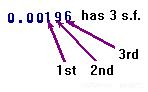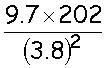HELP MATHS APPROXIMATING AND CHECKING CALCULATIONS Return Significant figures The 1st significant figure (s.f.) is the first digit which is not zero. The 2nd, 3rd, 4th,... significant figures follow on after the 1st digit. They may or not may be zeros. EXAMPLES:ROUNDING To round a number to a given number of significant places apply the same rule as with decimal places: if the digit is 5 or more, round up. EXAMPLES:Estimates and approximations Estimating is a good way of checking answers: Round the numbers to "easy" numbers, usually numbers with 1 or 2 significant figures. Work out the estimate using these easy numbers. For multiplying or dividing never approximate a number to zero (use 0.1, 0.01, 0.001,...) EXAMPLE: Laura does the calculationLaura's answer is 1356.9 Estimate:Laura's answer is not the right order of magnitude. It is 10 times too big.